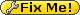• English only

# Lab for Automated Reasoning and Analysis LARA

## Complexity of the verification process

### Preliminary remarks

#### Terminology

• We say that two patterns have the same signature if, without their (optional) guard, they are identical.
• The set of pattern is assumed to be the cartesian product representing the set of inputs that a pattern would match.

#### Number of different positions

The maximum number of different positionswhich can be found in a given pattern matching expressionis naturally linear in terms of the size of the source code (whether you want to measure this as tokens, elements of the AST or characters is irrelevant).

#### Computing the set corresponding to a pattern can be done in linear time

…in terms of the size of the source code, that is. This should be obvious…

#### Consistency of the dimensions of the cartesian products

For every pattern, we build a cartesian product representing the set of inputs which it would match. By construction, the dimension of these products is always the same since it corresponds to. In particular, this means that taking the union of two “pattern sets” will yield yet another cartesian product with the same cardinality.

Note that we now also have:The proof is straightforward, but still requires that the dimensions match, otherwise the intersection could be empty for this other reason.

### Translation of the assumptions/axioms

We will always use unary relations to represent sets (and therefore set membership):will be translated to, andto.

For each statement which we want to prove or disprove, we make use of the user-provided informations about properties on the class hierarchy and the extractors.

These properties are always in one of the following forms:

• Set of extractors covering a given type:• Sealed and abstract classes:• Disjointness of extractors:This translates to:

••• andrespectively.

### Verifying disjunction

Recall that disjunction is verified for each pair of patterns. There are two options:

1. Either the patterns have different signatures, in which case we ignore their guards and verify that the interesection of their sets is empty…
2. …or they have the same signature, in which case we simply verify that the conjunction of their guards is false.

Since we limit our boolean expressions to QFBAPA (do we?), the formula for verifying the disjointness of two guardsand, namelyis itself in QFBAPA and its satisfiability can hence be checked with an algorithm in NP . This solves the second case.

The first case is not much harder. We can use the abovementioned equivalence for the intersection of cartesian products of same dimensions, and we need to insert only one quantifier to transform it to the desired form:

•…is translated to:

•Note that checking the satifiability should be doable in polynomial time, as all needs to be done is find one pair of sets among a finite list which are disjoint (and this information can only come from the assumptions/axioms, which are themselves in a finite number. (: I know this doesn't sound convincing.. let's do better).

We can also rely on the fact that we introduced only one quantifier and that the size of the generated formula is linear in terms of the size of the original one. This formula is hence inand checking its validity can hence be done in NP time.

### Verifying completeness/reachability

As an introductory remark, note that checking for reachability of a pattern p is equivalent to checking that the preceding patterns do not form a complete set over the values matched by p. With this in mind, observe that all formulas for checking completeness and reachability are in the form:When checking completeness, the setis the domain of the scrutinee, when checking reachability, it is the set of the observed pattern. (Note that this is in fact checking subsumption. Proper reachability checking would include verifying that the pattern set is a subset of the scrutinee set, but this does not introduce any additional complexity.) Also note that the sets over which the union is taken correspond to patterns which satisfy one of the following properties:

• the pattern has no guard
• the pattern has a guard, but there exists a set of patterns with the same signature such that the disjunction of their guards is equivalent to the true statement

Again, checking that second property on a disjunction of guardscan be done in NPTIME.

Usingquantifiers, we translate the previous (meta-)formula to:Along with the axioms and with proper renaming of variables, we get a formula in the decidable prefix class..

() Now I'm not so sure.. I'd like to say that it's in fact in, and that for a given pattern matching expressionis fixed, so the satisfiability of the underlying class is in NP, but I'm not sure that's allowed.. Is the fact that we can easily put a bound onfor a given expression enough to say it's a fixed constant? Since it's dependant on the input, I would not think so..

 Quantifier Free Boolean Algebra with Presburger Arithmetic is NP-Complete, technical report somewhere IIRC.

© EPFL 2018 - Legal notice